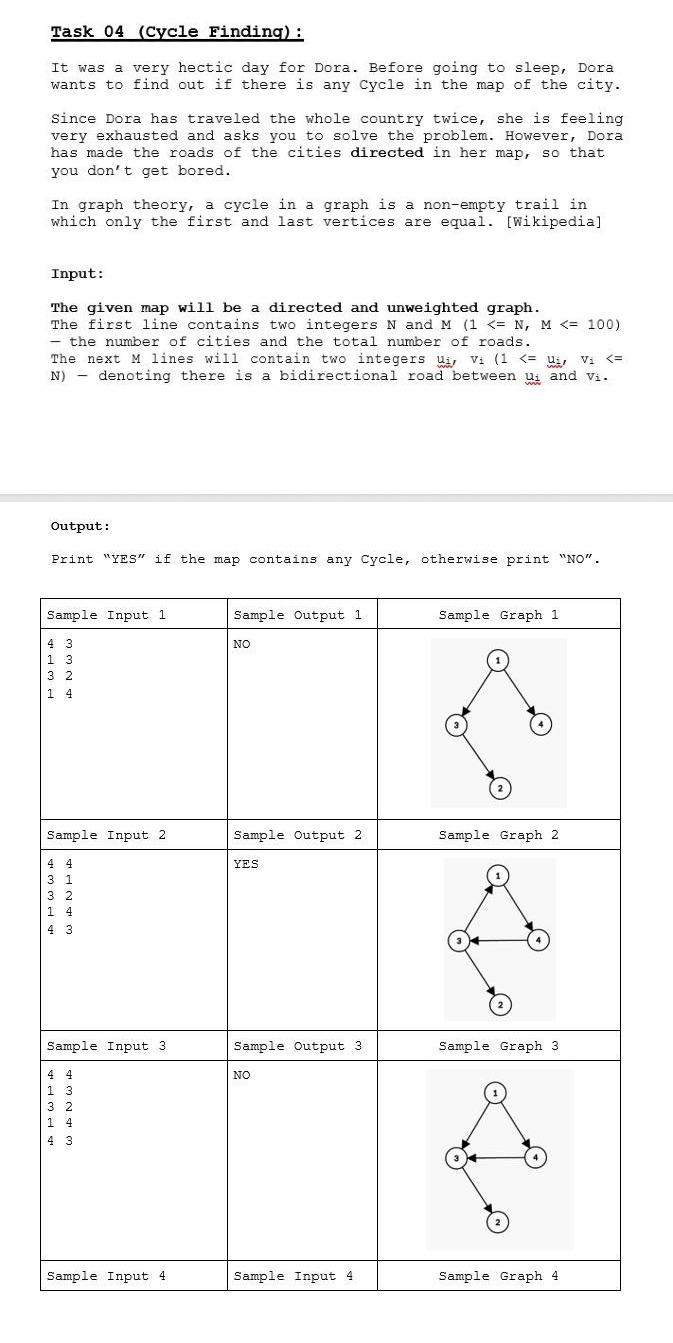# Question must use python   Task 04 (Cycle Finding): It was a very hectic day for Dora. Before going to sleep, Dora wants to find out if there is any cycle in the map of the city. Since Dora has traveled the whole country twice, she is feeling very exhausted and asks you to solve the problem. However, Dora has made the roads of the cities directed in her map, so that you don't get bored. In graph theory, a cycle in a graph is a non-empty trail in which only the first and last vertices are equal. [Wikipedia] Input: The given map will be a directed and unweighted graph. The first line contains two integers $$N$$ and $$M(1<=N, M<=100)$$ - the number of cities and the total number of roads. The next $$M$$ lines will contain two integers $$u_{i}, v_{i}\left(1<=u_{i}, v_{i}<=\right.$$ N) - denoting there is a bidirectional road between $$u_{i}$$ and $$v_{i}$$. Output: Print "YES" if the map contains any CYcle, otherwise print "NO".ZHX33I The Asker · Computer Science

must use pythonTranscribed Image Text: Task 04 (Cycle Finding): It was a very hectic day for Dora. Before going to sleep, Dora wants to find out if there is any cycle in the map of the city. Since Dora has traveled the whole country twice, she is feeling very exhausted and asks you to solve the problem. However, Dora has made the roads of the cities directed in her map, so that you don't get bored. In graph theory, a cycle in a graph is a non-empty trail in which only the first and last vertices are equal. [Wikipedia] Input: The given map will be a directed and unweighted graph. The first line contains two integers $$N$$ and $$M(1<=N, M<=100)$$ - the number of cities and the total number of roads. The next $$M$$ lines will contain two integers $$u_{i}, v_{i}\left(1<=u_{i}, v_{i}<=\right.$$ N) - denoting there is a bidirectional road between $$u_{i}$$ and $$v_{i}$$. Output: Print "YES" if the map contains any CYcle, otherwise print "NO".
More
Transcribed Image Text: Task 04 (Cycle Finding): It was a very hectic day for Dora. Before going to sleep, Dora wants to find out if there is any cycle in the map of the city. Since Dora has traveled the whole country twice, she is feeling very exhausted and asks you to solve the problem. However, Dora has made the roads of the cities directed in her map, so that you don't get bored. In graph theory, a cycle in a graph is a non-empty trail in which only the first and last vertices are equal. [Wikipedia] Input: The given map will be a directed and unweighted graph. The first line contains two integers $$N$$ and $$M(1<=N, M<=100)$$ - the number of cities and the total number of roads. The next $$M$$ lines will contain two integers $$u_{i}, v_{i}\left(1<=u_{i}, v_{i}<=\right.$$ N) - denoting there is a bidirectional road between $$u_{i}$$ and $$v_{i}$$. Output: Print "YES" if the map contains any CYcle, otherwise print "NO".